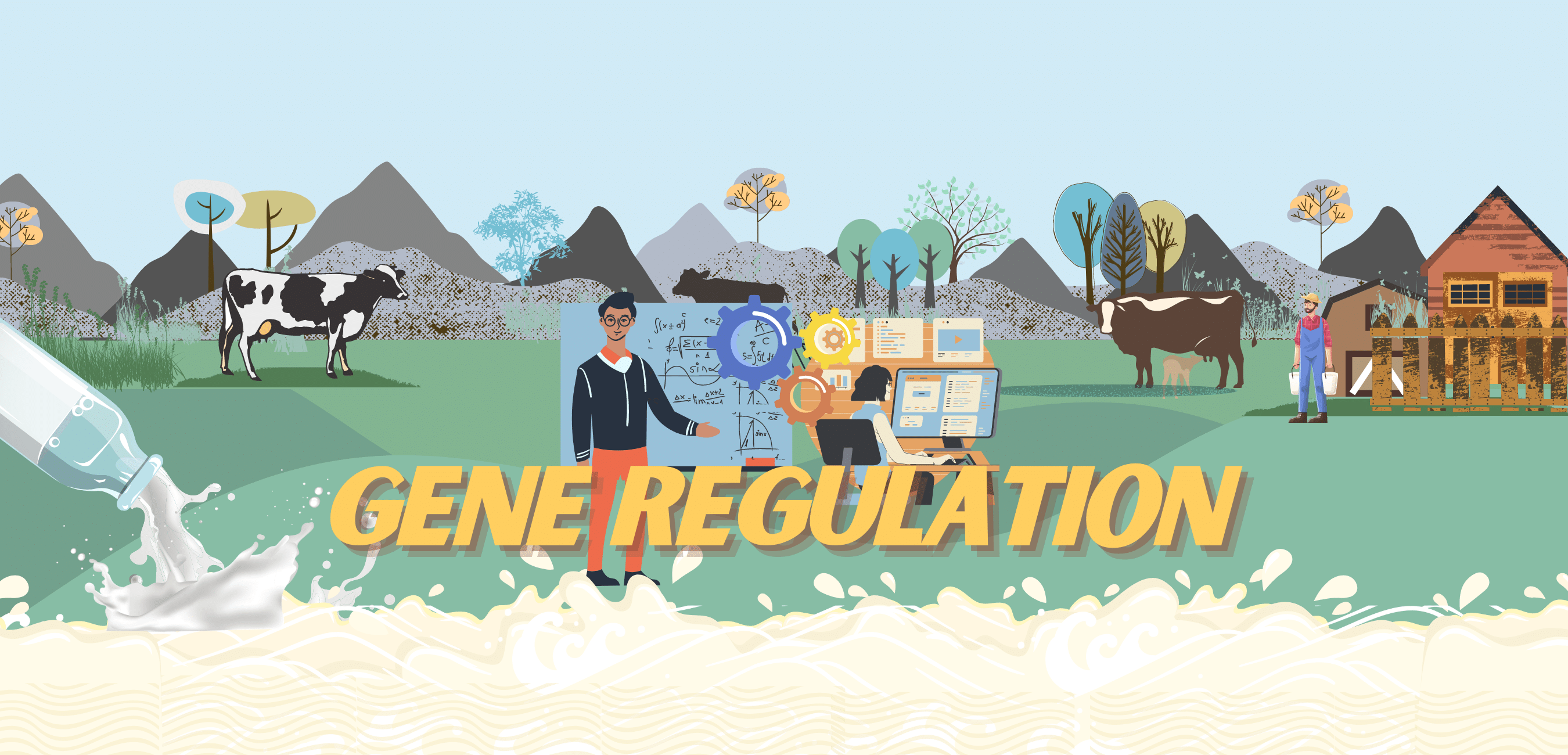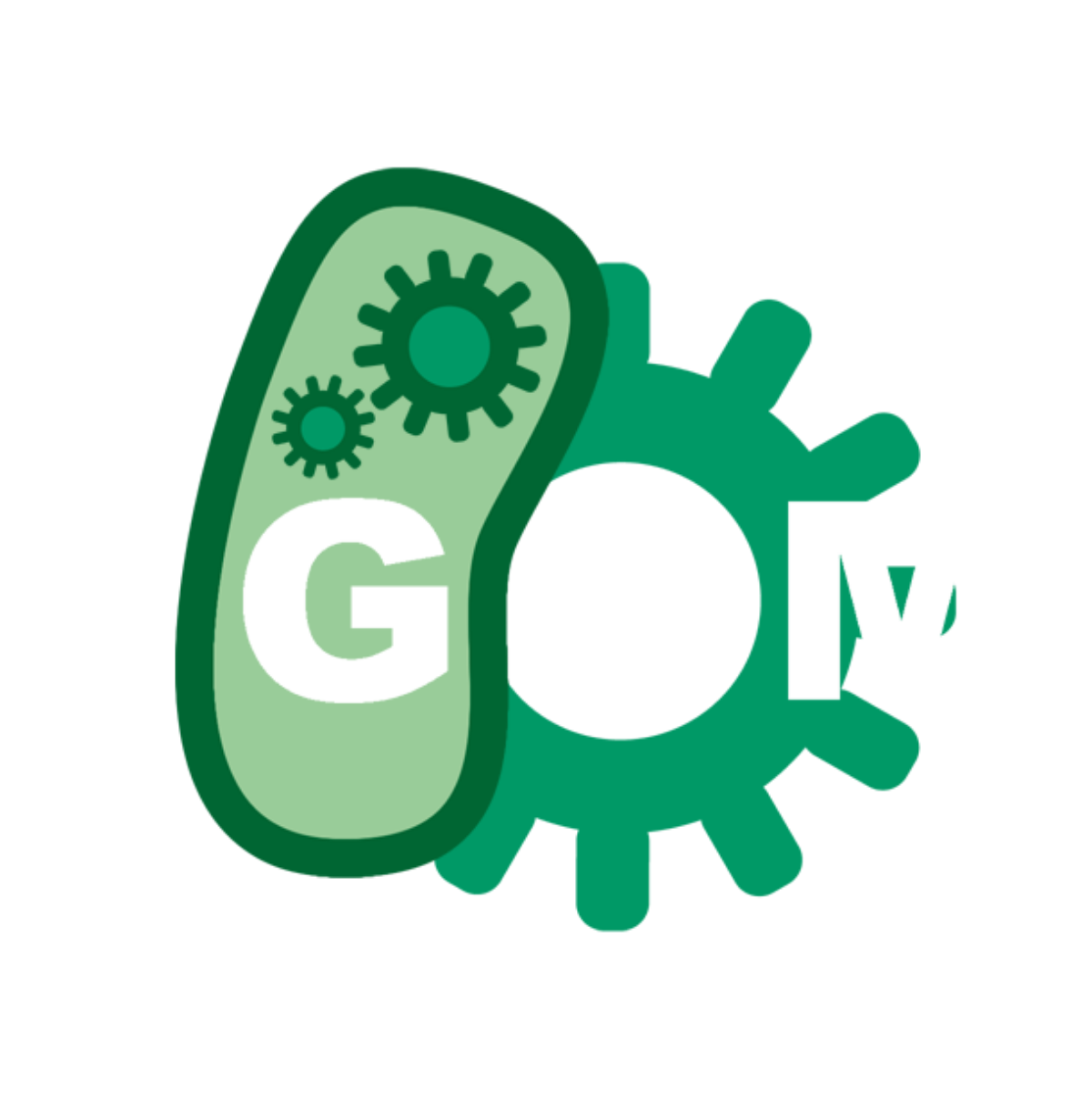# Team:IISER Kolkata/GeneRegulationLoading . . .Introduction

The following mathematical model tracks the variation of these following chemical species over time -AIP-I released by the pathogen Streptococcus Aureus, AgrA, AgrC, Phosphorylated AgrA, AgrC-AIP-I complex,DNaseI, NisinPV and LysisE7 . This is achieved by formulating ordinary differential equations for the rate of change of these chemical species. We also take into account rate of change of the three mRNA's in the system owing to the presence of three promoters. With this model we try to visualise how effective the model organism is at producing NisinPV, DNaseI and LysisE7 and the time scale of their production and accumulation .

Assumptions
• Time taken for transcription of these genes and translation of mRNA is negligible.
• The reaction follows Michaelis Menten kinetics and thereby follows the assumptions that come with it such as steady state, free ligand and rapid equilibrium approximation.
• the initial cocnentrations of all chemicals are zero except of AIP-I, AgrA and AgrC
• The transcription rate of mRNA's of P1, P2 and P3 promoter genes are assumed to be similar
• There are n model organisms in the system with a cell volume of $$\nu$$
Differential equations
$$\frac{d[AgrC]}{dt}=\alpha_c n K_{api} \frac{\frac{[AgrA-P]}{n\nu}}{k_{api}+frac{[AgrA-P]}{n\nu}}+ \alpha_c n K_b -\gamma_c[AgrC]-K_1[AgrC][AIP-I]+K_2[AgrC-AIP-I]$$ $$\frac{d[AIP-I]}{dt}=K_1[AgrC][AIP-I]+K_2[AgrC-AIP-I]$$ $$\frac{d[AgrA]}{dt}=\alpha_a n K_{api} \frac{\frac{[AgrA-P]}{n\nu}}{k_{api}+frac{[AgrA-P]}{n\nu}}+ \alpha_a n K_b-\gamma_a[AgrA]+K_{AP}[AgrA-P]-\frac{K_A}{n\nu}[AgrA][AgrC-AIP-I]$$ $$\frac{d[AgrC-AIP-I]}{dt}=K_1[AgrC][AIP-I]-\frac{K_A}{n\nu}[AgrA][AgrC-AIP-I]-K_2[AgrC-AIP-I]-D_1[AgrC-AIP-I]$$ $$\frac{d[AgrA-P]}{dt}=\frac{K_A}{n\nu}[AgrA][AgrC-AIP-I]- K_{AP}[AgrA-P] -D_2[AgrA-P]$$ $$\frac{d[M_2]}{dt}=n K_{api} \frac{\frac{[AgrA-P]}{n\nu}}{k_{api}+frac{[AgrA-P]}{n\nu}}+n K_b -\gamma_{M_{2}}M_2$$ $$\frac{d[M_3]}{dt}=n K_{api} \frac{\frac{[AgrA-P]}{n\nu}}{k_{api}+frac{[AgrA-P]}{n\nu}}-\gamma_{M_{3}}M_3$$ $$\frac{d[DNaseI]}{dt}=\alpha_D n K_{api} \frac{\frac{[AgrA-P]}{n\nu}}{k_{api}+frac{[AgrA-P]}{n\nu}}-\gamma_D[DNaseI]$$ $$\frac{d[NisinPV]}{dt}=\alpha_N n K_{api} \frac{\frac{[AgrA-P]}{n\nu}}{k_{api}+frac{[AgrA-P]}{n\nu}}-\gamma_N[NisinPV]$$ $$\frac{d[LysisE7]}{dt}=\alpha_L n K_{api} \frac{\frac{[AgrA-P]}{n\nu}}{k_{api}+frac{[AgrA-P]}{n\nu}}-\gamma_L[LysisE7]$$

The constant values used are tabulated below. Most of these values are taken from scientific literature and for those whose parameter values aren't available we have made educated approximations.

Parameter Values

Variable Dimension Description Initial Value
AgrC $$\mu$$ mol Total amount of un-complex AgrC in the population 0.1
AgrC-AIP-I $$\mu$$ mol Total amount of complex between AgrC and AIP 0
AgrA $$\mu$$ mol Total amount of un-phosphorylated AgrA in the population 0.1
AgrA-P $$\mu$$ mol Total amount of phosphorylated AgrA in the population 0
M_2 $$\mu$$ mol Total amount of RNA_2 in the population 0
M_3 $$\mu$$ mol Total amount of RNA_3 in the population 0
NisinPV $$\mu$$ mol Total amount of NisinPV in the population 0
DNaseI $$\mu$$ mol Total amount of DNaseI in the population 0
LysisE7 $$\mu$$ mol Total amount of LysisE7 in the population 0
n Number of cells in the E.coli population 0
$$\nu$$ $$\mu$$m^3 Volume of E.coli 1
AIP-I $$\mu$$ mol.$$\mu$$ m^3 Concentration of AIP in the environment
K_1 $$\mu$$ mol^-1.$$\mu$$ m^3.hour^-1 Association rate of complex AgrC-AIP-I 1
K_2 hour^-1 Dissociation rate of complex AgrC-AIP-I 0.1
K_A $$\mu$$ mol^-1.$$\mu$$ m^3.hour^-1 Phosphorylation rate of AgrA 10
K_AP hour^-1 Dephosphorylation rate of AgrA 1
D_1 hour^-1 Degradation rate of complex AgrC-AIP-I 2
D_2 hour^-1 Degradation rate of phosphorylated AgrA 2
$$\gamma$$_M_2 hour^-1 Degradation rate of RNA_2 2
$$\gamma$$_M_3 hour^-1 Degradation rate of RNA_3 2
$$\gamma$$_A hour^-1 Degradation rate of un-phosphorylated AgrA 2
$$\gamma$$_C hour^-1 Degradation rate of un-complex AgrC 2
$$\gamma$$_N hour^-1 Degradation rate of NisinPV 2
$$\gamma$$_D hour^-1 Degradation rate of DNaseI 2
$$\gamma$$_L hour^-1 Degradation rate of LysisE7 2
K_api $$\mu$$molhour^-1 Maximal AgrA dependent transcription rate of P2/P3 10
k_api $$\mu$$mol$$\mu$$m^3 Concentration of phosphorylated 1 AgrA required for half-maximal AgrA dependent transcription rate of P2/P3 1
K_b $$\mu$$molhour^-1 Basal transcription rate of P2 0.1
_A Effective factor of AgrA protein synthesis 1
$$\alpha$$_C Effective factor of AgrC protein synthesis 1
$$\alpha$$_N Effective factor of NisinPV protein synthesis 1
$$\alpha$$_L Effective factor of Lysi 1
Simulation and Results
• In figure A, we observe that the concentration of AIP-I secreted by pathogen streptococcus Aureus decreases steadily with time. This is because this AIP-I continuously binds to the AgrC present in our model organism and since the eventual release of NisinPV and DNaseI kills off S. Aureus, there is no way AIP-I in the system can be replenished. As a result, we see a nearly exponential drop in AIP-I concentration in the system.
• In figure B, the logarithm of the concentration of AIP-I in the system sees a linear decline which confirms that the concentration drop is indeed exponential.
• In figure C, we see a rapid increase in Nisin PV concentration within the first two time units and then a gradual decrease. The rapid increase, in the beginning, is because Nisin PV accumulates in the GMO and has no means to exit and kill the pathogen. Once there is enough Lysis E7 buildup in the cell, the GMO cell membrane ruptures releasing the nisin PV into the environment. This is then used up to eliminate the pathogen and hence we see a decreasing trend.
• In figure D, the logarithm of the concentration of Nisin PV sees a sharp rise and maintains around a particular threshold value.
• In figure E, the sharp rise in Lysis E7 concentration is due to accumulation in the GMO. Once Lysis E7 breaks through the cell wall and is released, concentration decreases. But we see sudden surges indicating that release isn’t gradual and at certain instances lysis gets accumulated before it is used to break down the cell membrane. But eventually, its concentration keeps decreasing with time.
• In figure F, the concentration of DNaseI follows the same trend as that of NisinPV since they are regulated by the same promoter and are released at the same time into the surrounding environment after significant Lysis E7 buildup and then is used for killing S.Aureus. Hence we see a quick significant rise and then a gradual fall.
• In figure G, the logarithm of the concentration of DNaseI sees the same trend as the logarithmic scale of NIsinPV concentration. The logarithm of the concentration of LysisE7 sees a similar trend but has a few kinks in the “threshold’ region owing to the sudden spikes in concentration as discussed earlier.
• In figure H, it is observed that the amount of Nisin PV increases with the amount of AIP-I. We see a sharp decline after a certain concentration of AIP-I because there is an excess of AIP-I in the system and most of NisinPV is used up in killing the pathogens which are high in number (inferred from an excess of AIP-I).
Sensitivity Analysis

Inference

From both plots, we infer that a higher initial concentration of AIP-I in the system results in an increase in the maximum NisinPV concentration. This is because .ore AIP-I can bind to AgrC forming more phosphorylated AgrA which binds to the promoter regulating Nisin PV. This leads to more NisinPV being produced overall and therefore the maximum NisinPV concentration in the system increases.

### References

• A Simulation of Synthetic agr System in E.coli ,Xiangmiao Zeng, Ke Liu, Fangping Xie, Ying Zhang, Lei Qiao, Cuihong Dai,Aiju Hou, and Dechang Xu,School of Food Science & Engineering Harbin Institute of Technology, P.R.C